# Design Series-Fed Patch Antenna for 5G Base Station Antenna

This example shows how to design and analyse the series fed patch antenna array at 28 GHz. The antenna has 64 elements arranged in 8x8 array configuration which is used as 5G mobile base station antenna at 28 GHz. The phased array steers its beam along the horizontal axis to provide coverage in different directions.### Create Variables

Create the variables and assign the values as per .

```Wp = 3.539e-3; Wc = 0.494e-3; Lc = 3.539e-3; Wm = 2.727e-3; Lm = 0.6269e-3; Wf = 0.72e-3; Lf = 2.215e-3;```

### Create Geometry

Use the `antenna.Rectangle` shape object to create all the rectangles in the structure and then join them. Visualize the structure using the `show` function.

```a = antenna.Rectangle('Length',Lf,'Width',Wf,'Center',[Lf/2,0]); transVec = Lf; for i =1:7 b = antenna.Rectangle('Length',Wp,'Width',Wp,'Center',[transVec+Wp/2,0]); transVec = transVec+Wp; c = antenna.Rectangle('Length',Lc,'Width',Wc,'Center',[transVec+Lc/2,0]); transVec = transVec+Lc; a = a+b+c; end last = antenna.Rectangle('Length',Wp,'Width',Wp,'Center',[transVec+Wp/2,0]); lastsub = antenna.Rectangle('Length',Lm,'Width',Wm,'Center',[transVec+Lm/2,0]); last = last-lastsub; conn = antenna.Rectangle('Length',Lc,'Width',Wc,'Center',[transVec+Lc/2,0]); a = a+last+conn; figure; show(a)```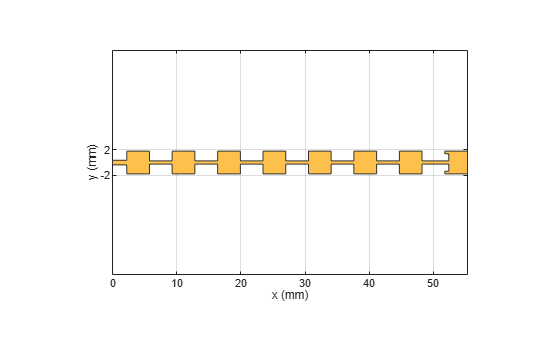### Design PCB Board

Use the `pcbStack` object to create the PCB stack. Create the ground plane and dielectric layers, and set the `BoardShape` to be the same shape as the ground plane. Visualize the PCB stack..

```ant = pcbStack; gnd = antenna.Rectangle('Length',57e-3,'Width',10e-3,'Center',[57e-3/2,0]); d = dielectric('Teflon'); d.EpsilonR = 2.2; ant.Layers={a,d,gnd}; ant.BoardShape = gnd; ant.BoardThickness = 0.254e-3; ant.FeedDiameter = Wf/2; ant.FeedLocations = [0,0,1,3]; figure; show(ant)```### Analyze Array Performance

Use the `mesh` function to manually change the mesh and set the `MaxEdgeLength` property to 0.4 mm to ensure there are 30 triangles per wavelength.

```figure; mesh(ant,'MaxEdgeLength',0.4e-3);```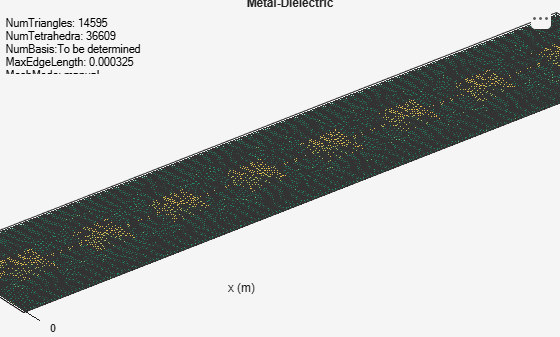Use the `memoryEstimate` function to calculate the memory required to solve the structure.

`memEst = memoryEstimate(ant,28e9)`
```memEst = '38 GB' ```

As the memory requirement is very high, you will need more time to calculate the S-parameters and plot the antenna pattern. This example includes a MAT file containing the return loss and pattern data computations. The code used to compute the results is available in the Return Loss and Pattern Data Computations section. On the machine with 64 GB of RAM and Intel(R) Xeon(R) W-2133 CPU processor it takes around 50 minutes to solve one frequency.

Load the MAT file and plot the return loss using the `rfplot` function.

```load seriesPatchData.mat figure; rfplot(out);```Use the `patternCustom` to plot the 2-D or 3-D radiation pattern of the antenna.

```phi = az'; theta = (90-el); MagE = pat'; figure; patternCustom(MagE,theta,phi);```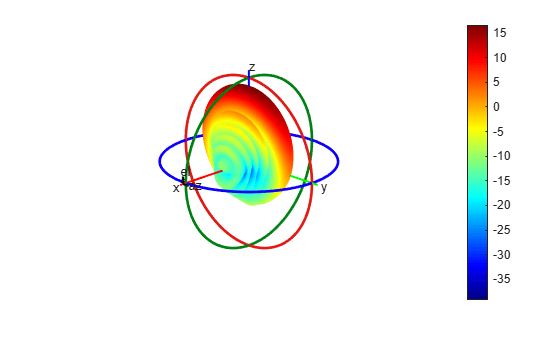### Plot Radiation Pattern Using Pattern Multiplication

Use pattern multiplication to plot the radiation pattern of the 8-by-8 array instead of solving the entire structure using the MoM solver. You will need a license to the Phased Array System Toolbox™ to use pattern multiplication.

Use the `phased.CustomAntennaElement` System object™ to build the custom antenna element using the pattern data from the MAT file loaded above.

```antenna = phased.CustomAntennaElement; antenna.FrequencyVector = [0 28e9]; antenna.AzimuthAngles = az; antenna.ElevationAngles = el; antenna.MagnitudePattern = pat; antenna.PhasePattern = zeros(size(pat));```

Use the `phased.ULA` System object™ to create the 8-by-8 array. Specify the custom element as the array element. Set the number of elements to 8, and set the element spacing to 3.5 mm.

```array = phased.ULA; array.Element = antenna; array.NumElements = 8; array.ElementSpacing = 3.5e-3;```

#### Pattern Multiplication

Use the `pattern` function to plot the 3-D radiation pattern.

```figure; pattern(array,28e9)```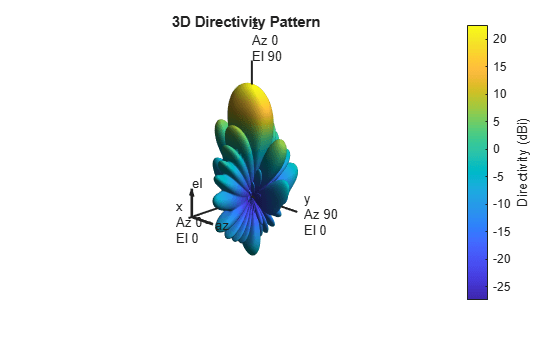Use the `pattern` function to plot the radiation pattern in the E-plane

```pat1 = pattern(array,28e9,0,0:1:90,'CoordinateSystem','rectangular'); pat2 = pattern(array,28e9,180,0:1:90,'CoordinateSystem','rectangular'); data1 = [pat1;flip(pat2)]; figure,plot(data1); grid on; xlabel('Theta(deg)'); ylabel('Magnitude(dB)'); title('E-Plane');```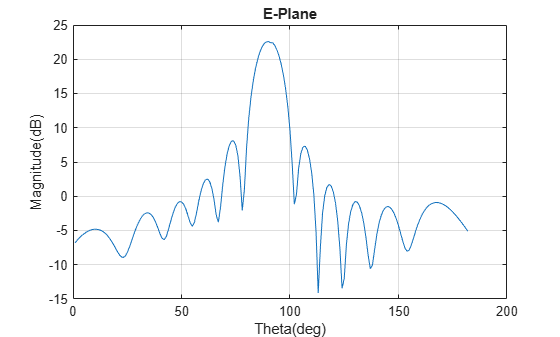Use the `pattern` function to plot the radiation pattern in the H-plane

```pat3 = pattern(array,28e9,90,-90:1:90,'CoordinateSystem','rectangular'); data2 = [pat3;flip(pat3)]; figure,plot(data2); grid on; xlabel('Theta(deg)'); ylabel('Magnitude(dB)'); title('H-Plane');```### Return Loss and Pattern DataComputations

```% out = sparameters(ant,linspace(27.5e9,28.5e9,11)); % figure; % rfplot(out); % figure, % pattern(ant, 28e9) % figure; % impedance(ant,linspace(27.5e9,28.5e9,11));```

### References

 “EM Simulation of 28 GHz Series-Fed Patch Antenna Array for 5G | 2019-02-01 | Microwave Journal.” Accessed January 21, 2022. https://www.microwavejournal.com/articles/31731-em-simulation-of-28-ghz-series-fed-patch-antenna-array-for-5g.

 Ishfaq, Muhammad Kamran, Tharek Abd Rahman, Yoshihide Yamada, and Kunio Sakakibara. “8×8 Phased Series Fed Patch Antenna Array at 28 GHz for 5G Mobile Base Station Antennas.” In 2017 IEEE-APS Topical Conference on Antennas and Propagation in Wireless Communications (APWC), 160–62. Verona, Italy: IEEE, 2017. https://doi.org/10.1109/APWC.2017.8062268.